Manipulating SurveyCTO Timestamps using Pandas | Outline India

## Manipulating SurveyCTO Timestamps using Pandas

#### By Gurjot Singh MahiHey guys! In this blog post, we will take a step-by-step tour to learn how we can manipulate the timestamps using the Python data analysis library - Pandas. Pandas is a fast, flexible, and easy to use open-source data analysis and manipulation tool built on top of the Python programming language.

At Outline India (hereafter, OI), we extensively use the SurveyCTO data collection platform to code our paper-based tools in digital format to conduct various field surveys. After each completed survey, OI researchers use the raw dataset file (in .csv document format) with multiple variables to perform the analysis using Pandas. Every raw dataset generated using SurveyCTO contains 3 mandatory timestamp variables – Submission Date, start time, and end time.

In this blog post, we will enquire about the various steps involved, from installing the pandas to carrying out the need-based data-time manipulation using in-built timestamp functions in the Pandas library.

So let's get started.

Step 1: Install the Pandas and Tabulate package

Before you go any further, make sure you have installed the Python and PIP package. Carry out the following command on the windows default command-line interpreter to install the Pandas and tabulate package,

Step 2: Import the Pandas and Tabulate library

We will import the Pandas and tabulate library and use this to read the raw dataset file and perform manipulations,

Step 3: Read the dataset file with timestamps

Now, it's time to read the raw dataset (.csv file) using Pandas to perform the various timestamp manipulations.

This gives us the output as follows,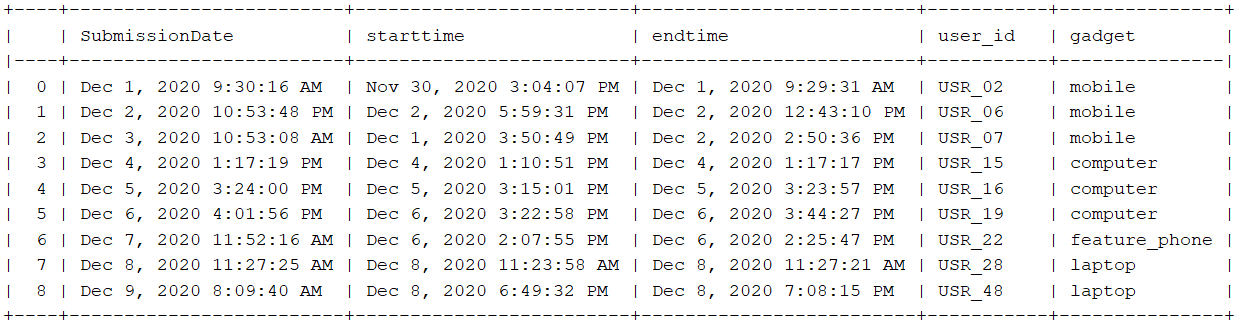As we can see in the output, the mentioned timestamp columns are in string format. To read the timestamp columns in DateTime type, we need to parse the mentioned columns using `parse_dates` parameter while reading the file.

The code will give us the following output: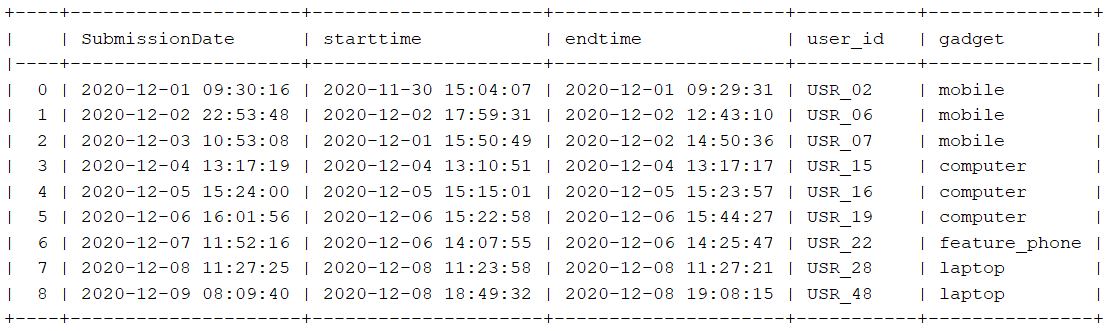Step 4: Perform timestamp manipulations

We can now perform any manipulation on the given timestamp columns based on our need.

a) Remove time from the timestamp
We can remove the time from the timestamp columns using,

The code will remove the time from the submission date column.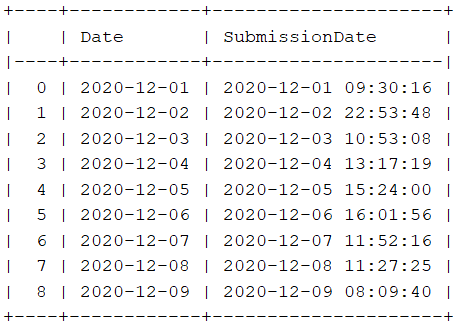b) Select rows with a specific date

Suppose we want to select the rows with greater or less than a particular date. We will use the inbuild loc function, which helps access a group of rows and columns by label(s) and logical operations.

As we can see from the following output, rows with a date greater than 2020-12-04 in the Date column are selected.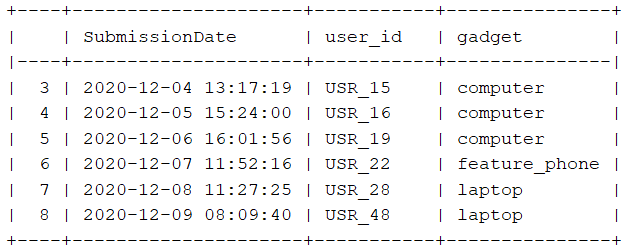If we want to select the rows within some particular start and end date, this can be done using the following code:

We get the given output,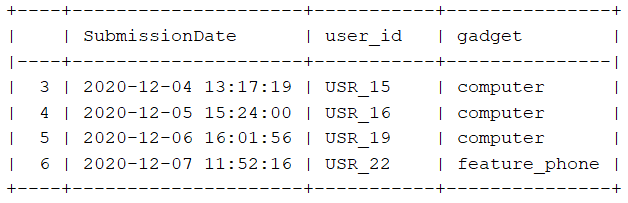c) Converting date to respective day name

The pandas give us the functionality to convert the date into the particular day name. We use the dt.day_name() function to convert the date into the respective day.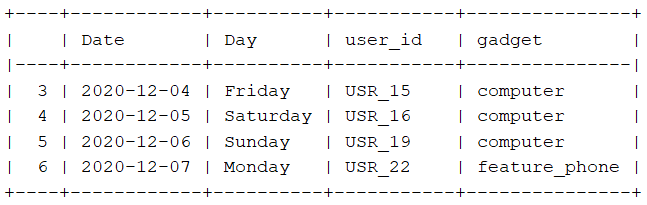d) Increase date by days, months, and years

The Pandas offset function provides the functionality to increase the date by days, months, and years.

This gives the output as,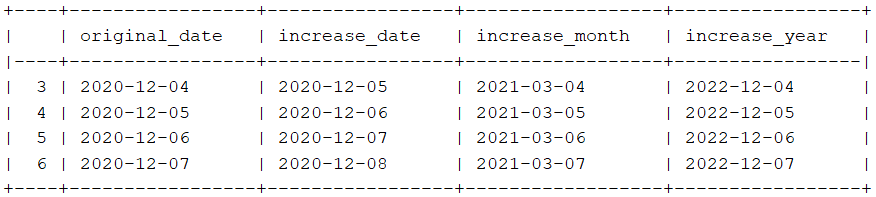We can also perform the date manipulation based on the day name. If we want the date to increase by 2, if the current day is Saturday and increase by one if it's Sunday.

This gives the following output: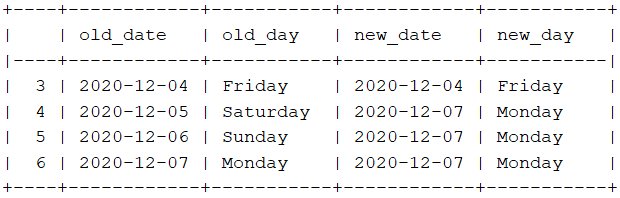e) Extract only time from the timestamp

Now let's talk about time manipulation. If we want to extract the time from the timestamp column, we can use the dt.time function just like date extraction performed in the last sections.

This gives us the following output: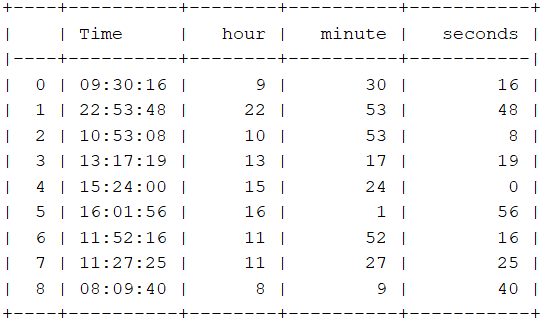f) Increase time by Hours, Minutes, and Seconds

Pandas .tseries functionality can be used to increase the Hours, Minutes, and Seconds in the timestamps.

This gives the output as,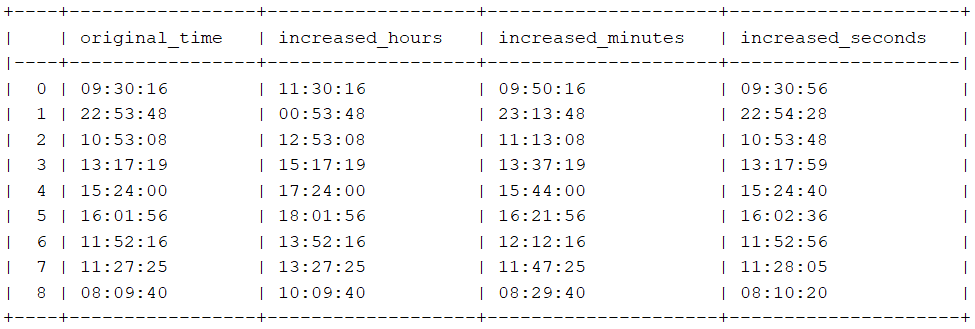ENDNOTES

So, folks, That's all for this blog. This article was written as the first of a series using Pandas to manipulate the timestamps. More are in line. Thank you if you kept reading until the end.

Complete code can be assessed at